One note: I’ll use a purely right-handed convention for coordinate systems. Right-handed means that if you hold your right hand with your fingers curled and the thumb sticking out, the thumb points along the Z axis and the fingers point in the direction of rotation from the X axis to the Y axis, as shown in Figure 50.2. Rotations about an axis are counter-clockwise, as viewed looking down an axis toward the origin. The handedness of a coordinate system is just a convention, and left-handed would do equally well; however, right-handed is generally used for object and world space. Sometimes, the handedness is flipped for view space, so that increasing Z equals increasing distance from the viewer along the line of sight, but I have chosen not to do that here, to avoid confusion. Therefore, Z decreases as distance along the line of sight increases; a view space coordinate of (0,0,-1000) is directly ahead, twice as far away as a coordinate of (0,0,-500).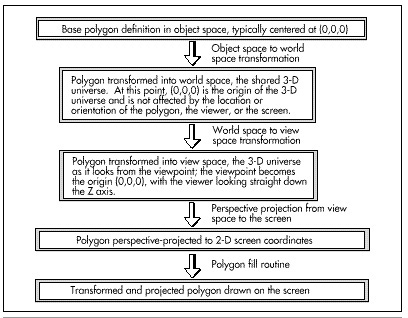Figure 50.1
The 3-D drawing pipeline.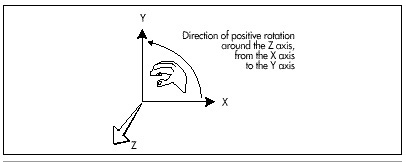Figure 50.2
A right-handed coordinate system.

Projection

Working backward from the final image, we want to take the vertices of a polygon, as transformed into view space, and project them to 2-D coordinates on the screen, which, for projection purposes, is assumed to be centered on and perpendicular to the Z axis in view space, at some distance from the screen. We’re after visual realism, so we’ll want to do a perspective projection, in order that farther objects look smaller than nearer objects, and so that the field of view will widen with distance. This is done by scaling the X and Y coordinates of each point proportionately to the Z distance of the point from the viewer, a simple matter of similar triangles, as shown in Figure 50.3. It doesn’t really matter how far down the Z axis the screen is assumed to be; what matters is the ratio of the distance of the screen from the viewpoint to the width of the screen. This ratio defines the rate of divergence of the viewing pyramid—the full field of view—and is used for performing all perspective projections. Once perspective projection has been performed, all that remains before calling the polygon filler is to convert the projected X and Y coordinates to integers, appropriately clipped and adjusted as necessary to center the origin on the screen or otherwise map the image into a window, if desired.

Translation

Translation means adding X, Y, and Z offsets to a coordinate to move it linearly through space. Translation is as simple as it seems; it requires nothing more than an addition for each axis. Translation is, for example, used to move objects from object space, in which the center of the object is typically the origin (0,0,0), into world space, where the object may be located anywhere.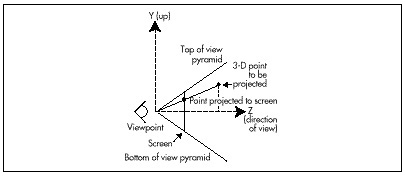Figure 50.3
Perspective projection.

Rotation

Rotation is the process of circularly moving coordinates around the origin. For our present purposes, it’s necessary only to rotate objects about their centers in object space, so as to turn them to the desired attitude before translating them into world space.

Rotation of a point about an axis is accomplished by transforming it according to the formulas shown in Figure 50.4. These formulas map into the more generally useful matrix-multiplication forms also shown in Figure 50.4. Matrix representation is more useful for two reasons: First, it is possible to concatenate multiple rotations into a single matrix by multiplying them together in the desired order; that single matrix can then be used to perform the rotations more efficiently.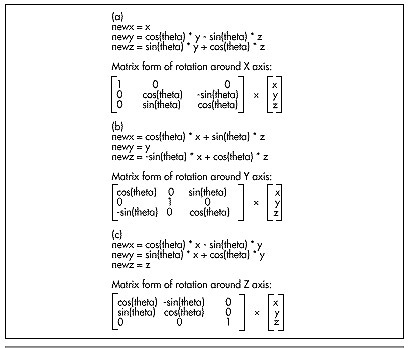Figure 50.4
3-D rotation formulas.

Second, 3×3 rotation matrices can become the upper-left-hand portions of 4×4 matrices that also perform translation (and scaling as well, but we won’t need scaling in the near future), as shown in Figure 50.5. A 4×4 matrix of this sort utilizes homogeneous coordinates; that’s a topic way beyond this book, but, basically, homogeneous coordinates allow you to handle both rotations and translations with 4×4 matrices, thereby allowing the same code to work with either, and making it possible to concatenate a long series of rotations and translations into a single matrix that performs the same transformation as the sequence of rotations and transformations.

There’s much more to be said about transformations and the supporting matrix math, but, in the interests of getting to working code in this chapter, I’ll leave that to be discussed as the need arises.

A Simple 3-D Example

At this point, we know enough to be able to put together a simple working 3-D animation example. The example will do nothing more complicated than display a single polygon as it sits in 3-D space, rotating around the Y axis. To make things a little more interesting, we’ll let the user move the polygon around in space with the arrow keys, and with the “A” (away), and “T” (toward) keys. The sample program requires two sorts of functionality: The ability to transform and project the polygon from object space onto the screen (3-D functionality), and the ability to draw the projected polygon (complete with clipping) and handle the other details of animation (2-D functionality).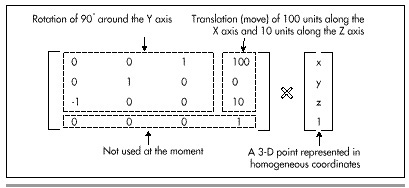Figure 50.5
A 4×4 Transformation Matrix.

Happily (and not coincidentally), we put together a nice 2-D animation framework back in Chapters 47, 48, and 49, during our exploratory discussion of Mode X, so we don’t have much to worry about in terms of non-3-D details. Basically, we’ll use Mode X (320×240, 256 colors), and we’ll flip between two display pages, drawing to one while the other is displayed. One new 2-D element that we need is the ability to clip polygons; while we could avoid this for the moment by restricting the range of motion of the polygon so that it stays fully on the screen, certainly in the long run we’ll want to be able to handle partially or fully clipped polygons. Listing 50.1 is the low-level code for a Mode X polygon filler that supports clipping. (The high-level polygon fill code is mode independent, and is the same as that presented in Chapters 38, 39, and 40, as noted further on.) The clipping is implemented at the low level, by trimming the Y extent of the scan line list up front, then clipping the X coordinates of each scan line in turn. This is not a particularly fast approach to clipping—ideally, the polygon would be clipped before it was scanned into a line list, avoiding potentially wasted scanning and eliminating the line-by-line X clipping—but it’s much simpler, and, as we shall see, polygon filling performance is the least of our worries at the moment.

Graphics Programming Black Book © 2001 Michael Abrash